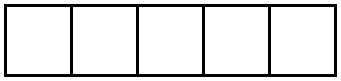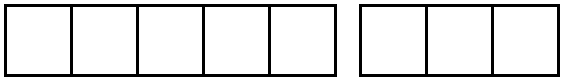# Fraction Operations

## Objective

Decompose non-unit fractions less than or equal to 2 as a sum of unit fractions, as a sum of non-unit fractions, and as a whole number times a unit fraction.

## Common Core Standards

### Core Standards

?

• 4.NF.B.3.B — Decompose a fraction into a sum of fractions with the same denominator in more than one way, recording each decomposition by an equation. Justify decompositions, e.g., by using a visual fraction model. Examples: 3/8 = 1/8 + 1/8 + 1/8 ; 3/8 = 1/8 + 2/8 ; 2 1/8 = 1 + 1 + 1/8 = 8/8 + 8/8 + 1/8.

• 4.NF.B.4.A — Understand a fraction a/b as a multiple of 1/b. For example, use a visual fraction model to represent 5/4 as the product 5 × (1/4), recording the conclusion by the equation 5/4 = 5 × (1/4).

?

• 3.NF.A.1

• 3.NF.A.2

## Criteria for Success

?

1. Decompose a fraction less than or equal to 2 into a sum of unit fractions, recording the decomposition with an equation.
2. Decompose a fraction less than or equal to 2 into a sum of fractions with the same denominator in more than one way, recording each decomposition with an equation.
3. Decompose a fraction less than or equal to 2 into a multiple of unit fractions, recording the decomposition with an equation.
4. Use these decompositions to show why a fraction greater than one is equivalent to a mixed number and vice versa.
5. Justify decompositions with a visual model, such as a tape diagram or number line.

## Tips for Teachers

?

• In Teaching Student-Centered Mathematics, 3-5, vol.2, John A. Van de Walle states, "The term ‘improper’ can be a source of confusion because it implies that this representation is not acceptable, which is false. Instead it is often the preferred representation in algebra. Avoid using this term and instead use ‘fraction’ or ‘fraction greater than one’” (p. 217). Thus, this lesson and all following avoid the use of the term with students.
• Before the Problem Set, you could have students play around with manipulatives to decompose fractions into a sum of unit fractions or non-unit fractions, similar to Joe Schwartz’s blog post “Building Towers." If you don’t have fraction towers or even fraction tiles, you can create them from paper.

#### Fishtank Plus

• Problem Set
• Student Handout Editor
• Vocabulary Package

?

### Problem 1

During Lesson 1, Francisco and Harry were playing with their fraction strips.

Part A

They decomposed the whole as follows:What fraction of the whole is each piece? What fraction is represented in the entire model?

Part B

Then, Francisco and Harry were given a different shape. They decomposed it as follows:What fraction of the whole is each piece? What fraction is represented in the entire model? Explain your thinking.

### Problem 2

1. Represent the following fractions as a sum of unit fractions, as a multiple of a unit fraction, and in at least one other way. Write your answer as an equation and justify your equation with a tape diagram or number line.

${{5\over3}}$

1. Use your work in part (a) to show why ${{{5\over3}}}={1{2\over3}}$.

### Problem 3

1. Represent the following fractions as a sum of unit fractions, a sum of non-unit fractions, as a multiple of a unit fraction, and in at least one other way. Write your answer as an equation and justify your equation with a tape diagram or number line.

${1{3\over8}}$

1. Use your work in part (a) to show why ${1{3\over8}}={11\over8}$.

## Discussion of Problem Set

?

• In #1–#4, why do we need to label 1 on our tape diagrams and number lines? What would happen if we didn’t?
• What do you notice about #5(a) and #5(c)?
• What is the advantage of representing fractions using multiplication?
• In our lesson, when we expressed ${{5\over3}}$ as ${\left(3\times{1\over3}\right)+\left(2\times{1\over3}\right)}$, what property were we using?

?

Decompose the following fractions (a) as a sum of unit fractions, (b) as a multiple of a unit fraction, and (c) in at least one other way.

1.  ${{7\over6}}$

2.  ${1{3\over4}}$

?